﻿ Fermat Collocation Method for Solvıng a Class of the Second Order Nonlinear Differential Equations

### Fermat Collocation Method for Solvıng a Class of the Second Order Nonlinear Differential Equations

Salih Yalçınbaş, Dilek TaştekinOPEN ACCESSPEER-REVIEWED

## Fermat Collocation Method for Solvıng a Class of the Second Order Nonlinear Differential Equations

Salih Yalçınbaş1,, Dilek Taştekin1

1Department of Mathematics Celal Bayar University, Muradiye, Manisa, Turkey

### Abstract

In this paper, a matrix method based on collocation points on any interval [a,b] is proposed for the approximate solution of some second order nonlinear ordinary differential equations with the mixed conditions in terms of Fermat polynomials. The method, by means of collocation points, transforms the differential equation to a matrix equation which corresponds to a system of nonlinear algebraic equations with unknown Fermat coefficients. Also, the method can be used for solving Riccati equation. The numerical results show the effectiveness of the method for this type of equation. Comparing the methodology with some known techniques shows that the present approach is relatively easy and high accurate.

### At a glance: Figures

1
Prev Next

• Yalçınbaş, Salih, and Dilek Taştekin. "Fermat Collocation Method for Solvıng a Class of the Second Order Nonlinear Differential Equations." Applied Mathematics and Physics 2.2 (2014): 33-39.
• Yalçınbaş, S. , & Taştekin, D. (2014). Fermat Collocation Method for Solvıng a Class of the Second Order Nonlinear Differential Equations. Applied Mathematics and Physics, 2(2), 33-39.
• Yalçınbaş, Salih, and Dilek Taştekin. "Fermat Collocation Method for Solvıng a Class of the Second Order Nonlinear Differential Equations." Applied Mathematics and Physics 2, no. 2 (2014): 33-39.

 Import into BibTeX Import into EndNote Import into RefMan Import into RefWorks

### 1. Introduction

Nonlinear ordinary differential equations are frequently used to model a wide class of problems in many areas of scientific fields; chemical reactions, spring-mas systems bending of beams, resistor-capacitor-inductance circuits, pendelums, the motion of a rotating mass around another body and so forth [1, 2]. These equations have also demonstrated their usefulness in ecology and economics. Thus, methods of solution for these equations are of great importance to engineers and scientist. Although many important differential equations can be solved by well known analytical tecniques, a greater number of physically significant differential equations can not be solved [2, 3]. On the other hand, Riccati differential equations occur a very important class of nonlinear differential equations. These play a fundamental role in control theory; for example, optimal control, filtering and estimation, decompling and order reduction etc [4, 5].

In this paper, for our aim we consider the second order nonlinear ordinary differential equation of the form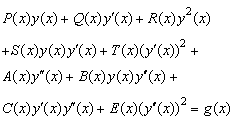(1)



under the mixed conditions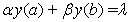(2)

and look for the approximate solution in the form(3)

[7, 8]

which is a Fermat polynomial of degree N, where yn(n = 0,1,,N)are the coefficients to be determined. Here P(x), Q(x), R(x), S(x), T(x), A(x), B(x), C(x), E(x) and g(x) are the functions defined on axb; the real coefficients α,β and λ are appropriate constants. Note that in the case of, S(x)=T(x)=A(x)=B(x)=C(x)=E(x)=0 in Eq. (1), it is a Riccati Equation [9, 10, 11]. Eq.(1) is a simple case of the general class of nonlinear ordinary differential equations. Despite its simplicity, the changes of obtaining an elementary solution are quite remote. It is well known that no general formula exists for the solution of the nonlinear ordinary differential equations, also of Eq.(1) moreover, certain special types of Eq.(1) having exact or approximate solutions may be solved by means of known methods [12, 13]. In the other cases, that is, for the second order nonlinear differential equations which can not be solved by known methods, the new Fermat collocation method to be presented in this paper can be used.

### 2. Fundamental Matrix Relations

Our aim is to find the matrix form of each term in the nonlinear equation given by Eq. (1). Firstly, we consider the solution y(x) defined by a truncated series (3) and then we can convert to the matrix form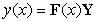(4)

where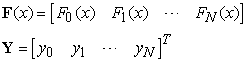If we differentiate expression (4) with respect to x, we obtain(5)

whereBy using (4), the matrix form of expression y2(x) is obtained as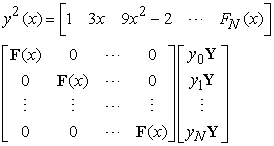or briefly(6)

where



By using the expression (4), (5) and (6) we obtain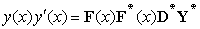(7)

Following a similar way to (6), we have(8)

where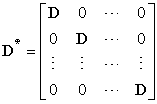Besides, applying a similar way to (4), (5) and (6), can be written, respectively(9)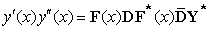(10)

and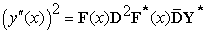(11)

where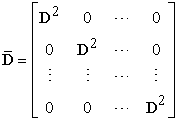### 3. Matrix Relations Based on Collocation Points

Let us use the collocation points defined by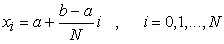(12)

in order to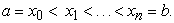By putting the collocation points (9) into Eq. (1), we get the equation(13)

By using the relations (4), (5), (6), (7), (8), (9), (10) and (11); the system (13) can be written in the matrix formor shortly(14)

where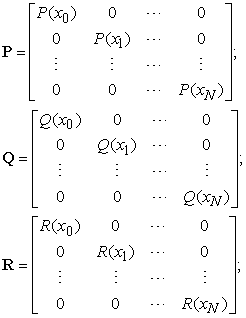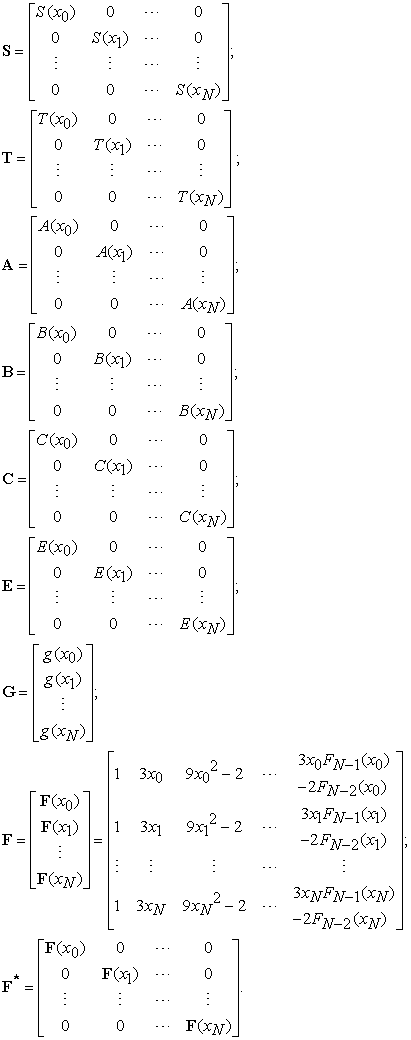### 4. Method of Solution

By substituting the matrix relations in section two, into matrix equation (14) we have the fundamental matrix equation clearlyor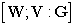(15)

where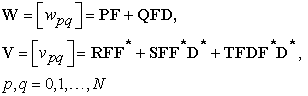We can find the corresponding matrix equation for the condition (2), using the relation (4), as follows: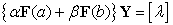(16)

so thatWe can write the corresponding matrix form (16) for the mixed condition (2) in the augmented matrix form as(17)

where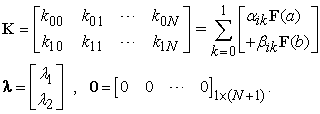To obtain the approximate solution of Eq. (1) with the mixed condition (2) in the terms of Fermat polynomials, by replacing the row matrix (17) by the last row of the matrix (14), we obtain the required augmented matrix: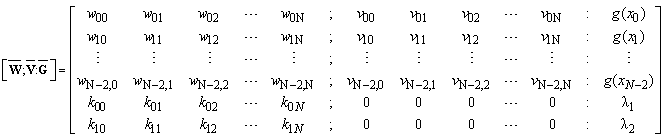or the corresponding matrix equation(18)

where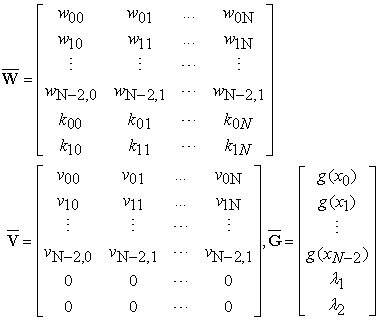The unknown coefficients set {y0,y1,…,yN} can be determined from the nonlinear system (18). As a result, we can obtain approximate solution in the truncated series form (3).

### 5. Accuracy of Solution

We can check the accuracy of the solution by following procedure [12, 13, 14]: The truncated Fermat series in (3) have to be approximately satisfying Eq. (1); that is, for each x=xi[a,b], i=1,2,and E(xi)10-ki (ki is any positive integer).

If max(10-ki)=10-k (k is any positive integer) is prescribed, then the truncation limit N is increased until the difference E(xi) at each of the points xi becomes smaller than the prescribed 10-k.

### 6. Numerical Examples

The method of this study is useful in finding the solutions of second-order nonlinear ordinary differential equations in terms of Fermat polynomials. We illustrate it by the following examples.

Example 6.1. Let us first consider the first-order nonlinear differential equation(19)

with conditionand the approximate solution y(x) by the truncated Taylor polynomial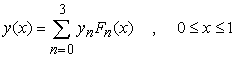whereFor N=3 the collocation points become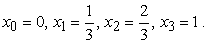From the fundamental matrix equations for the given equation and condition respectively are obtained as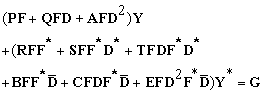and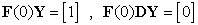so that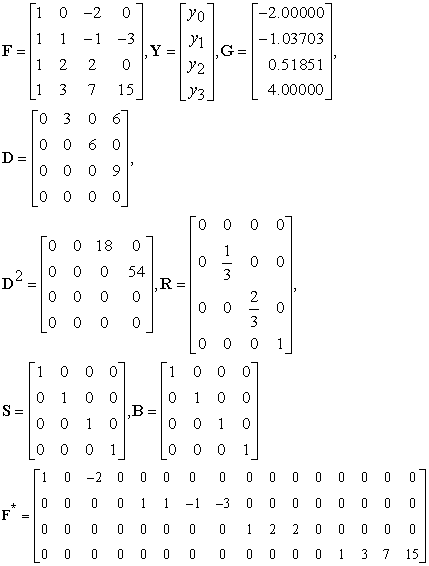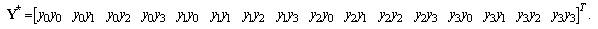The augmented matrix for this fundamental matrix equation is calculated.From the obtained system, the coefficients y0,y1,y2 and y3 are found as y0=5/3, y1=0, y2=1/3 and y3 =0.

Hence we have the Fermat polynomial solution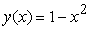Example 6.2. Consider the following nonlinear differential equation given by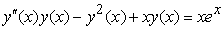(20)

with the initial conditionSo thatThe solutions obtained for N=3,5 are compared with the exact solution is ex, which are given in Figure 1.We compare the numerical solution and absolute errors for N=3,5 in Table 1.

#### Table 1. Comparison of the numerical errors of Example 6.2

Example 6.3. Let us first consider the second order nonlinear differential equation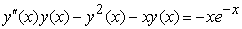(21)

with condition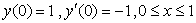Taking N=3,5 we obtain the approximate solution of this nonlinear differential equation.

The values of this solution are compared with the exact solution is ex and given errors in Table 2.

### 7. Conclusion

A new technique, using the Fermat polynomial, to numerically solve the second order nonlinear differential equations is presented. Nonlinear ordinary differential equations are usually difficult to solve analyticaly. Then it is required to obtain the approximate solutions. For this reason, the present method has been proposed for approximate solution and also analytical solution.

In this paper we have presented a suggested method to solve second order nonlinear ordinary differential equations with mixed conditions using the matrix method based on collocation points on any interval [a,b]. The matrix method avoids the difficulties and massive computational work by determining the analytic solution. Table 1 illustrates the errors obtained by using the procedure outlined above.

On the other hand, from Table 1, it may be observed that the errors found for different N show close agreement for various values of xi. Moreover, approximate solutions of Example 3 show very good agreement with the exact solution, and also in Example 1 we get the exact solution. Besides, tables show that closer the zero, better results are obtained. However, more term of the Fermat series is required for accurate calculation for large x.

A considerable advantage of the method is that Fermat coefficients of the solution are found very easily by using the computer programs. In addition, an interesting feature of this method is to find the analytical solutions if the equation has an exact solution that is a polynomial of N degree or less than N.

The method can also be extended to the high order nonlinear ordinary differential equations with variable coefficients, but some modifications are required.

### References

  G.F.Corliss, Guarented error bounds for ordinary differential equations, in theory and numeric of ordinary and partial equations (M.Ainsworth, J.Levesley, W.A Light, M.Marletta, Eds), Oxford Universty press, Oxford, pp. 342 (1995).In article  H. Bulut, D.J. Evens, on the solution of Riccati equation by the Decomposition method, Intern. J. Computer Math., 79(1) (2002) 103-109.In article CrossRef  G. Adomian, Solving frontier problems of physics the decomposition method, Kluwer Academic Publisher Boston, (1994).In article CrossRef  V. Hernandez, J.J. Ibonez, J. Peinodo, E. Arias, A GMRES-based BDF method for solving Differential Riccati Equations, Appl. Math. Comput. amc. 2007. 06. 021.In article  M. A. El-Tawil, A.A. Bahnasawi, A. Abdel-Naby, Solving riccati differential equation using adomian’s decomposition method, Appl. Math. Comput. 157 (2004) 503-514.In article CrossRef  D. Taştekin, S. Yalçınbaş, M. Sezer, Taylor collocation method for solving a class of the first order nonlinear differential equations, Mathematical and Computational Applications, vol.18, no.3, pp. 383-391, 2013.In article  A. F. Horadam, University of New England, Armidale, Australia, 1983.In article  L. Carliztz, Restricted Compositions, The Fibonacci Quarterly 14, 3, 254-264, 1976.In article  M. Sezer, A method for the approximate solution of the second order linear differential equations in terms of Taylor polynomials, International Journal of Mathematical Education in Science and Technology 27(6), 821-834, 1996.In article CrossRef  S. Yalçınbaş, Taylor polynomial solutions of nonlinear Volterra-Fredholm integral equations, Applied Mathematics and Computation 127, 195-206, 2002.In article CrossRef  S. Yalçınbaş, K. Erdem, Approximate solutions of nonlinear Volterra integral equation systems, International Journal of Modern Physics B 24(32), 6235-6258, 2010.In article CrossRef  L.M. Kells, Elemetary Differential Equations, McGraw-Hill Book Company, Newyork, (1965).In article  S.L. Ross, Differential Equations, John Wiley and Sons, Inc. Newyork, (1974).In article  R.A. Adams, Calculus, Addison-wesley Publisher, 562 (1990).In article